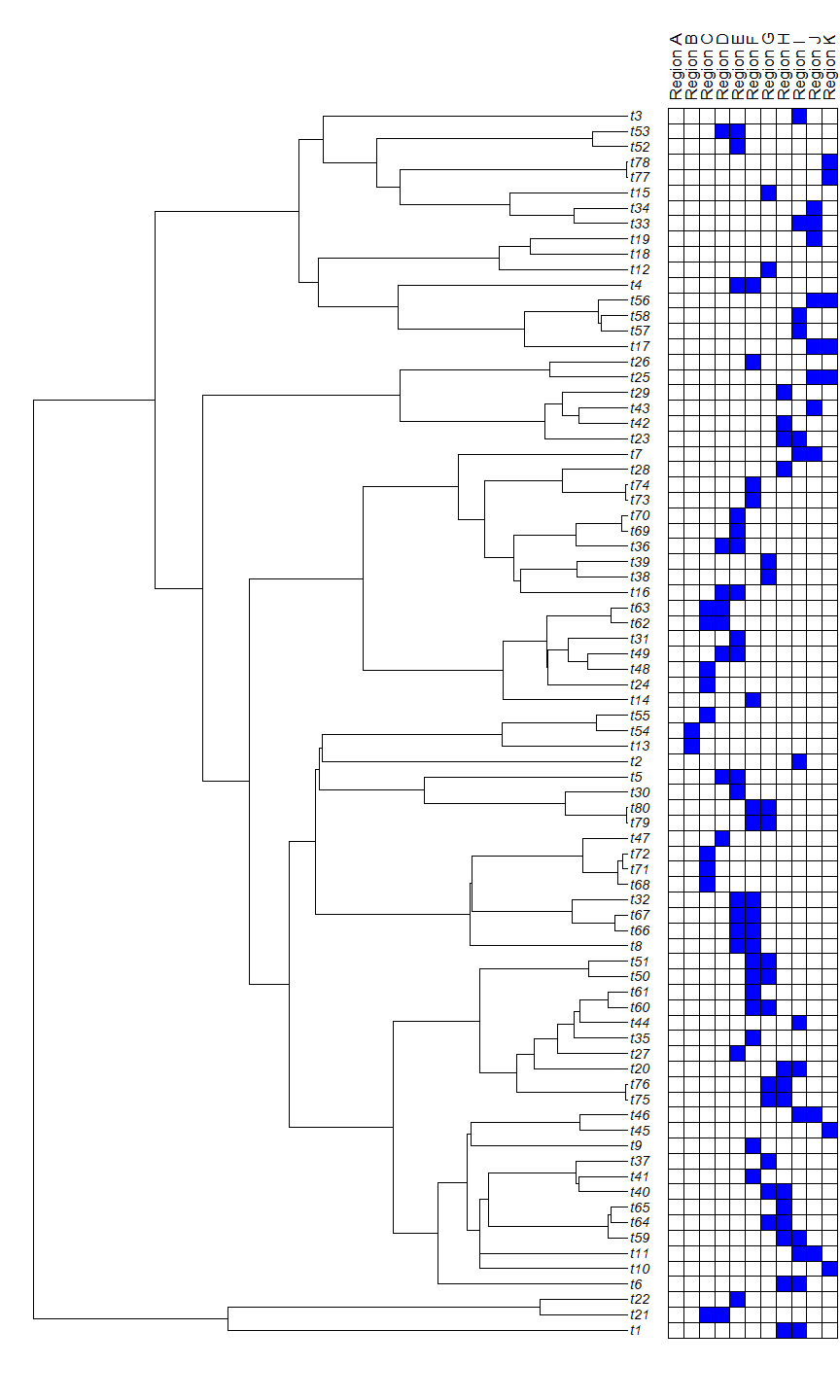## Sunday, February 11, 2018

### Plotting a tree with a grid of presence/absence geographic information at the tips in R

As a follow-up to the post I wrote yesterday about visually annotating a plotted tree with discrete states at the tips, the original poster asked how to apply the same approach to show presence absence from a geographic region. The following is my attempt at this.

First, I'm going to simulate some imaginary biogeographic data. These I will make simulate under a super-simple island model in which a species can occupy at most two regions, and to get from (say) region A to B, it must first go through A+B. This simulation looks as follows:

``````library(phytools)
## regions A:L
regions<-LETTERS[1:12]
states<-vector()
ind<-1
for(i in 1:length(regions)){
states[ind]<-regions[i]
if(i!=length(regions)){
states[ind+1]<-paste(regions[i],"&",regions[i+1],sep="")
ind<-ind+2
}
}
Q<-matrix(0,length(states),length(states),dimnames=list(states,states))
for(i in 1:(length(states)-1)){
Q[i,i+1]<-0.5
Q[i+1,i]<-0.5
}
tree<-pbtree(n=80,scale=60)
x<-sim.Mk(tree,Q)
X<-sapply(regions,function(r,x,zeros){
zeros[grep(r,x)]<-1
zeros },x=x,zeros=rep(0,Ntip(tree)))
rownames(X)<-tree\$tip.label
``````

More importantly, here is what the data look like:

``````tree
``````
``````##
## Phylogenetic tree with 80 tips and 79 internal nodes.
##
## Tip labels:
##  t1, t21, t22, t6, t10, t11, ...
##
## Rooted; includes branch lengths.
``````
``````head(X)
``````
``````##     A B C D E F G H I J K L
## t1  0 0 0 0 0 0 0 1 1 0 0 0
## t21 0 0 1 1 0 0 0 0 0 0 0 0
## t22 0 0 0 0 1 0 0 0 0 0 0 0
## t6  0 0 0 0 0 0 0 1 1 0 0 0
## t10 0 0 0 0 0 0 0 0 0 0 1 0
## t11 0 0 0 0 0 0 0 0 1 1 0 0
``````

in which `X` is a numeric 0/1 presence/absence matrix for my 12 regions.

Now we're ready to plot. For this I will simply adapt what I posted yesterday:

``````tree<-reorder(tree,"cladewise")
X<-X[tree\$tip.label,]
plotTree(tree,plot=FALSE)
obj<-get("last_plot.phylo",envir=.PlotPhyloEnv)
plotTree(tree,lwd=1,ylim=c(0,obj\$y.lim*1.05),xlim=c(0,obj\$x.lim*1.2),
ftype="off")
obj<-get("last_plot.phylo",envir=.PlotPhyloEnv)
h<-max(obj\$xx)
fsize<-0.6
for(i in 1:Ntip(tree)){
lines(c(obj\$xx[i],h),rep(obj\$yy[i],2),lty="dotted")
text(h,obj\$yy[i],tree\$tip.label[i],cex=fsize,pos=4,font=3,offset=0.1)
}
s<-max(fsize*strwidth(tree\$tip.label))
start.x<-1.05*h+s
cols<-setNames(c("white","blue"),0:1)
for(i in 1:ncol(X)){
text(start.x,max(obj\$yy)+1,paste("Region",colnames(X)[i]),pos=4,srt=90,
cex=0.7,offset=0)
for(j in 1:nrow(X)){
xy<-c(start.x,obj\$yy[j])
y<-c(xy-0.5,xy+0.5,xy+0.5,xy-0.5)
asp<-(par()\$usr-par()\$usr)/(par()\$usr-par()\$usr)*
par()\$pin/par()\$pin
x<-c(xy-0.5*asp,xy-0.5*asp,xy+0.5*asp,xy+0.5*asp)
polygon(x,y,col=cols[as.character(X[j,i])])
}
start.x<-start.x+asp
}
``````That's all there is to it.

1.Hi Liam,

Thanks very much for this review it has been really helpful! I have managed to plot the tree, and I've set up a matrix in the same format you have described with gene names. However, all boxes are white/empty and none are blue. The script doesn't cause an error - do you have any suggestions on why this may be the case?

I'm very new to R. Many thanks, Jess

1.Did you ever find a solution to this? I am having the same issue.

Note: due to the very large amount of spam, all comments are now automatically submitted for moderation.